# Fibonacci as a Trading ModelFibonacci is a great trading tool that stands the test of time and will never go out of fashion. It is based on a figure present in the universe called the "golden ratio". This concept is also valid in the financial markets. Read here about the Fibonacci trading model.

That mathematician was Leonardo of Pisa, better known as Fibonacci, and in his "Book of Calculus," Fibonacci promoted the new number system, demonstrating how simple it was compared to the Roman numerals that were used throughout Europe.Source: BBC

Fibonacci was called after Filius Bonacci or son of Bonacci, as they called his father. We must recognize in Fibonacci one of the first men who brought Arabic mathematics to Europe. In addition, he put the name of Greek mathematics very high and made it known among merchants. He was the one who was in charge of taking out the mathematics of the monasteries and the monopoly of the scholars.

Fibonacci explained the development of natural growth phenomena through its well-known numerical sequence. Wherever you find growth in nature, you will find Fibonacci numbers. The Fibonacci sequence is also the mathematical cousin of the golden number, a number that has haunted human culture for thousands of years.

Multibank Review
Capital.com Review
Markets.com Review

## How Does the Fibonacci Sequence Work?

This sequence is generated by adding two consecutive numbers to obtain the following:
f1 = f2 = 1
Fn = Fn - 1 + Fn - 2 for n> = 3

As a result of this formula, we have the Fibonacci series that corresponds to:

1 2 3 5 8 13 21 34 56 90 146 .....

However, the utility provided by this series lies in its fundamental properties, discovered in the 18th century:

1. If the numbers that are consecutive in the series are divided, that is, 1/1, 1/2, 2/3, 3/5, 5/8, 8/13, etc. It will be seen that the result obtained tends to the number 0.618.

2. If the non-consecutive numbers in the series are divided, that is, ½, 1/3, 2/5, 3/8, 5/13, 8/21, and so on. It will be observed that the result obtained tends to the number 0.382.

3. If the ratio of any number in the series to the next lowest number is now calculated, that is, 21/13, 13/8, 8/5 ... the result tends to 1.618, which is the inverse of 0.618.

4. If the ratio of any number in the series to the next lowest non-consecutive number is now calculated, that is, 8/21, 5/13, 8/3 ... the result tends to 2,618, which is the inverse of 0.382.

## How Do We Relate to the Fibonacci Series?

The idea behind this series is that the logarithmic spiral that can be built based on it describes part of the growth figure that can be seen throughout the Universe.

Examples of manifestation of this spiral are seen in the shell of the snail, the shape of the galaxy, the human ear, and many others.

Therefore, it is believed that the stock market, in addition to representing an example of the behavior of the human mass, is a manifestation of the phenomenon of natural growth that characterizes all human progress.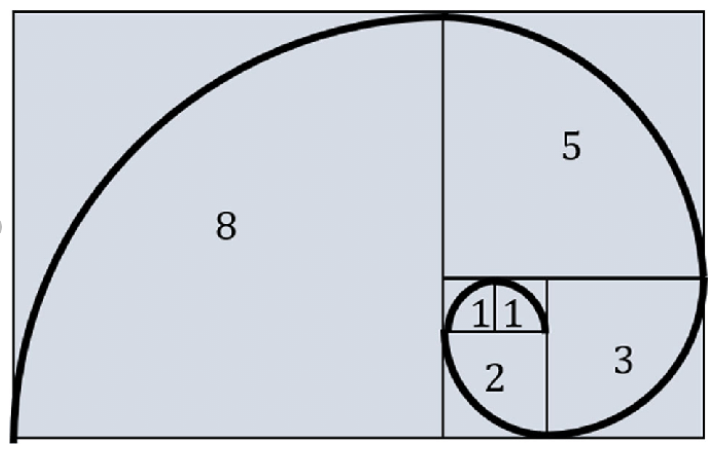The agents that participate in the market are in continuous search of tools that manage to give correct signals of purchase and sale to obtain superior returns.

The main objectives that the Fibonacci Series intends to achieve in its application to the stock and forex market are the following in our opinion:

• It seeks to predict price ranges, objectives that a financial instrument should reach when it is in a certain trend.
• It seeks to determine the period that a trend will last, and when a change will occur in it.

## Fibonacci Trading Model Using Time Zone

This technical analysis tool is a set of vertical lines that are drawn together by time intervals corresponding to the numbers of the Fibonacci sequence. The most relevant market events are said to occur through the time intervals corresponding to Fibonacci numbers 1, 2, 3, 5, 8, 13, 21, 34, etc.

The first line is placed at an extreme point on the chart and the lines that follow are placed at increasingly wider intervals according to the Fibonacci sequence. It consists of looking for significant changes in price near the vertical lines. Time bands are more applicable to long-term analysis of price action and are likely to be of limited value when studying short-term charts.

Consider this type of analysis only when looking at charts spanning several years and noting significant changes near the reference time-slot lines on the chart.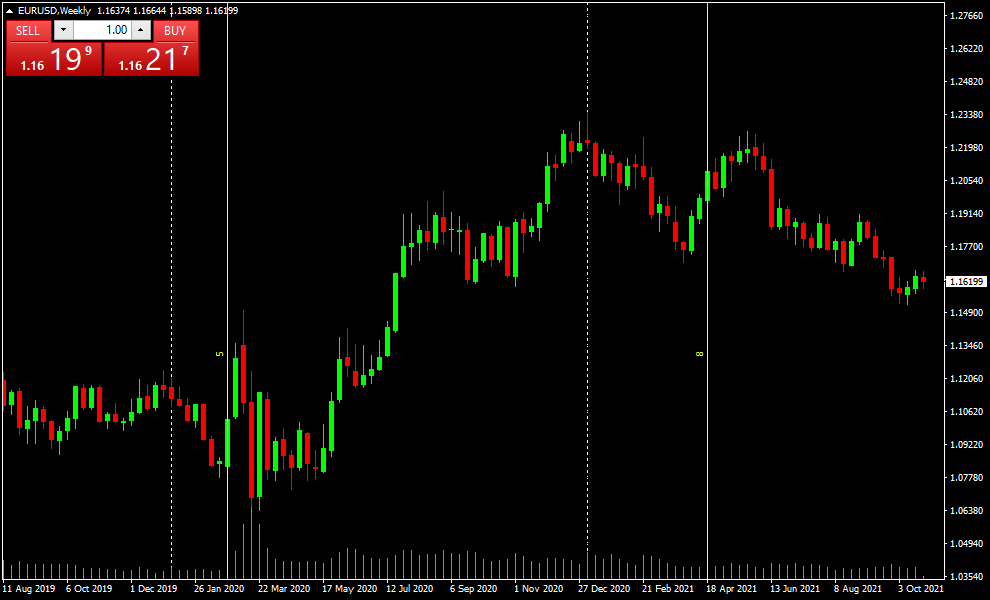## Fibonacci Trading Model Using Arcs

The radius of these arcs represents the distances along the line of movement the market makes in proportion to its length and is equivalent to the 38.2%, 50.0%, and 61.8% Fibonacci levels on the chart.

By interpreting Fibonacci arcs, you can anticipate price support and resistance.

Remember that because the arcs are circular about the chart axis, the points where they cross the price date will vary depending on the size of the chart scale.

It is important to view the arc zones in a larger environment that allows us to associate the number of candlesticks that have been held within the fibo zone or area to know how strong the break will be in the future.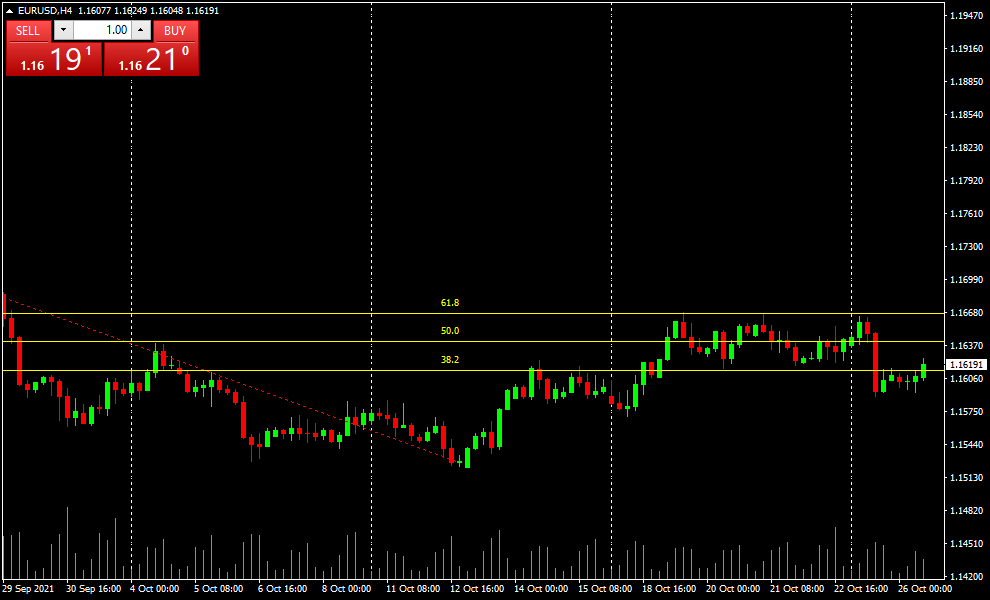## Fibonacci Trading Model Using Fans

Fibonacci fans are sets of sequential trendlines drawn from a trough or peak through a set of points dictated by Fibonacci retracements. To create them, a trader draws a trendline off of which to base the fan, usually covering the low and high prices of a security over a given period.

A charting technique consisting of three diagonal lines corresponding to Fibonacci ratios to help identify key support and resistance levels.

They are created by drawing a trend line through two points (usually from highs to lows in a given period) and then dividing the vertical distance between the two points by the Fibonacci ratios of 38.2%, 50%, and 61.8%.

We can use this as a trading model by determining a trading zone or channel within the Fibo trading ranges we deem appropriate.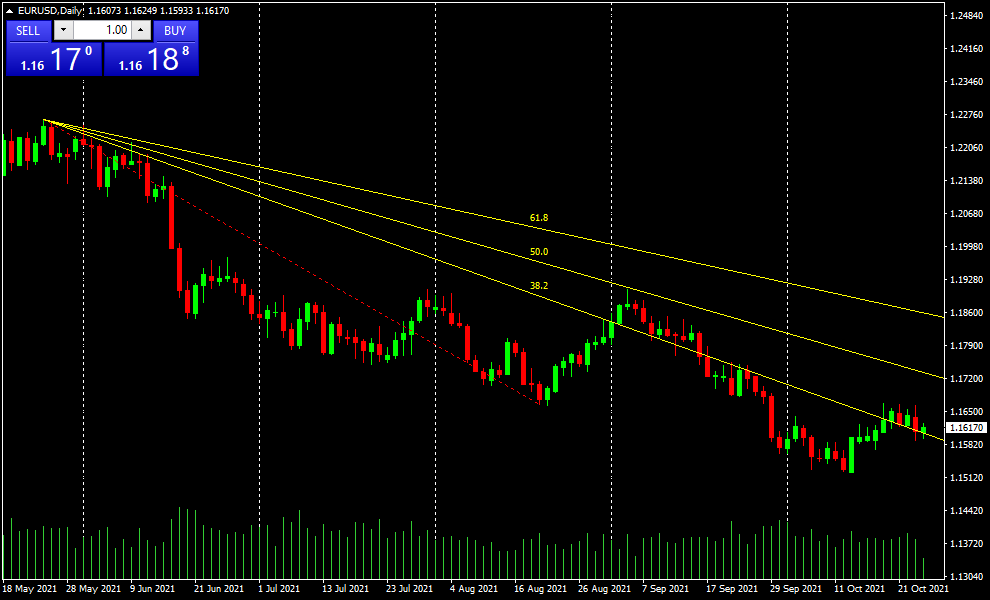## Fibonacci Trading Model Using Extensions

The Fibonacci expansion is used to determine expected resistances or supports following a trend. In other words, to determine the possible "stations" or "target zones" that the price will have following a confirmed trend.

The Fibonacci expansion is formed by two straight lines at 3 points. Then the possible resistances or target prices given by the following Fibonacci numbers are extrapolated (these three numbers are the most used): 61.8 - 100 - 161.8.

Generally, Fibonacci expansions find several temporary resistances, and we can emphasize that its main problem is to draw it since it is difficult to determine which minimum or maximum to use. It will be up to the trader to choose the one he considers more relevant in the information offered by the market.

We can operatively use this alternative using extension 1,618 as a take profit point.

Fibonacci spread levels are most accurate on the most popular and highly liquid currency pairs, as well as on stocks and futures contracts with a high level of trading.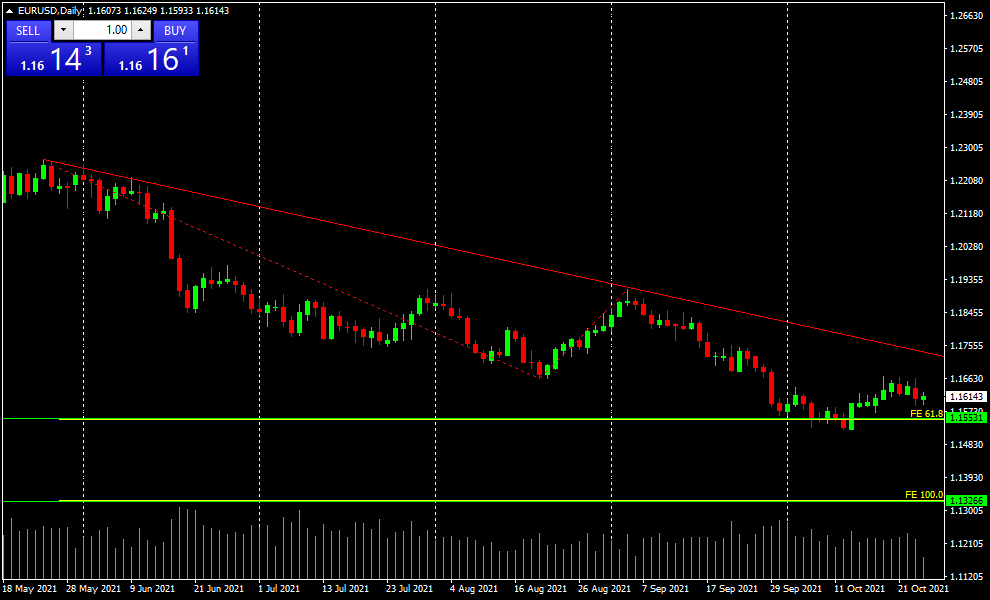## Fibonacci Trading Model Using Retracements

The second principle of technical analysis indicates that prices move in trends and that they can be bullish or bearish. Once a trend has shown sufficient signs of termination, either by a breakout or the confirmation of a trend reversal figure, the trader contemplates the possibility of a reversal in prices. A reversal represents a move in the opposite direction of the previous trend. It can take the form of a drop in price following an uptrend or a rebound in price following a downtrend.

The Fibonacci retracement is a very famous instrument that can be very useful as it can show inflection points with disconcerting accuracy. That is why it should be used in combination with other indicators to maximize the trading opportunity of a financial instrument. This method is used as part of a trend trading strategy by many traders.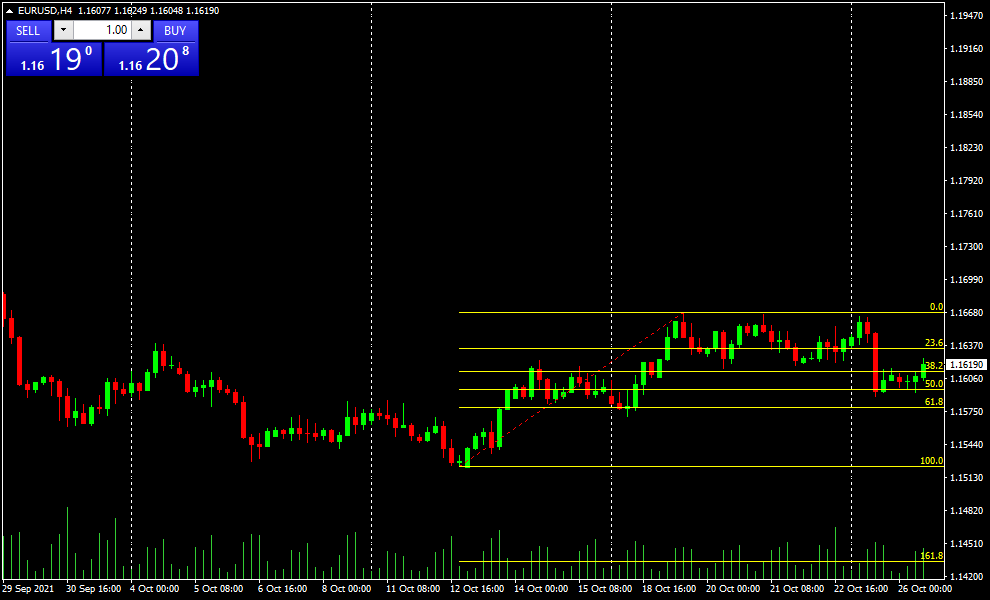Regardless of which Fibonacci tool you use, the important thing is always to correctly identify the relevant supports and resistances in the charts. Also, always use the major market trends.